Go Math Grade 8 Answer Key Chapter 6 Functions PDF is provided here for download. The best-loved math experts are provided an easy way of solving maths questions along with the explanations. Every problem is simplified with a clear explanation with many tricks. Great problems with a high level of difficulty also solved in an easy way. Refer to Go Math Grade 8 Answer Key Chapter 6 Functions now to begin your practice.

Prefer learning using Go Math Grade 8 Chapter 6 Functions Solution Key. To get the good results in an exam you must prepare with the Go Math Grade 8 Chapter 6 Answer Key. Use HMH Go Math Grade 8 Answer Key for the best practice of maths. Step by step explanation is provided for every question below. Build your confidence in solving math problems by practicing with Go Math Grade 8 Answer Key Chapter 6 Functions.

Lesson 1: Identifying and Representing Functions

Lesson 2: Describing Functions

Lesson 3: Comparing Functions

Lesson 4: Analyzing Graphs

Model Quiz

Mixed Review

### Guided Practice – Identifying and Representing Functions – Page No. 158

Complete each table. In the row with x as the input, write a rule as an algebraic expression for the output. Then complete the last row of the table using the rule.

Question 1.Type below:
_______________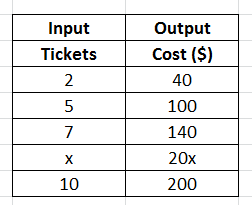Explanation:
Unit Cost of ticket = 40/2 = 20
Total cost = 20x where x is the number of tickets.
x = 20x
10 = 20(100) = 200

Question 2.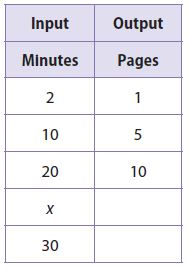Type below:
_______________Explanation:
Number of pages per minute = 1/2 = 0.5
Total cost = 0.5x where x is the number of minutes.
x = 0.5x
30 = 0.5(30) = 15

Question 3.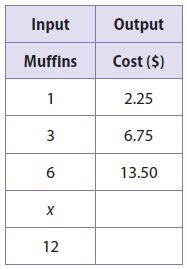Type below:
_______________Explanation:
Units cost of Muffins = 2.25/1 = 2.25
Total cost = 2.25x where x is the number of muffins
x = 2.25x
12 = 2.25(12) = 27

Determine whether each relationship is a function.

Question 4._______________

Function

Explanation:
Each input is assigned to exactly one output.

Question 5._______________

Not a function

Explanation:
The input value is 4 is paired with two outputs 25 and 35

Question 6.
The graph shows the relationship between the weights of 5 packages and the shipping charge for each package. Is the relationship represented by the graph a function? Explain.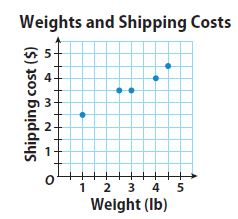_______________

Function

Explanation:
Each input is assigned to exactly one output.

Essential Question Check-In

Question 7.
What are four different ways of representing functions? How can you tell if a relationship is a function?
Type below:
_______________

The function can be represented by an equation, table, graph, and Venn diagram.
If a relationship is a function, each input is paired with exactly one output.

### Independent Practice – Identifying and Representing Functions – Page No. 159

Determine whether each relationship represented by the ordered pairs is a function. Explain.

Question 8.
(2, 2), (3, 1), (5, 7), (8, 0), (9, 1)
_______________

Function

Explanation:
Each input value is paired with exactly one output value.

Question 9.
(0, 4), (5, 1), (2, 8), (6, 3), (5, 9)
_______________

Not a function

Explanation:
The input value is 5 is paired with two outputs 1 and 9

Question 10.
Draw Conclusions
Joaquin receives $0.40 per pound for 1 to 99 pounds of aluminum cans he recycles. He receives$0.50 per pound if he recycles more than 100 pounds. Is the amount of money Joaquin receives a function of the weight of the cans he recycles? Explain your reasoning.
_______________

Yes

Explanation:
The amount of money increases with the weight of the cans. No weight will result in the same amount of money earned.

Question 11.
A biologist tracked the growth of a strain of bacteria, as shown in the graph.a. Explain why the relationship represented by the graph is a function.
Type below:
_______________

The relationship is a function as each input has been assigned exactly one output. There is only one number of bacteria for each number of hours.

Question 11.
b. What If?
Suppose there was the same number of bacteria for two consecutive hours. Would the graph still represent a function? Explain.
Type below:
_______________

Yes. If the number of bacteria for two consecutive hours is the same, one input will still be paired with one output, hence the relationship is still a function.

Question 12.
Multiple Representations
Give an example of a function in everyday life, and represent it as a graph, a table, and a set of ordered pairs. Describe how you know it is a function.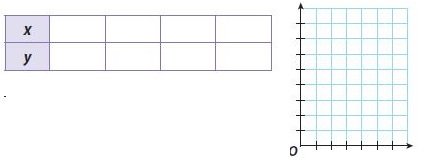Type below:
_______________

The cost of a bouquet of flowers and the number of flowers in the bouquet is a function. The unit cost of flowers = $0.85 and x the number of flowers. Hence, C= 0.85x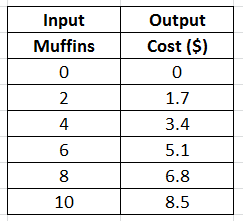(2, 1.7), (4, 3.4), (6, 5.1), (8, 6.8), (10, 8.5) Each value of the input is paired with exactly one output. ### Identifying and Representing Functions – Page No. 160 The graph shows the relationship between the weights of six wedges of cheese and the price of each wedge.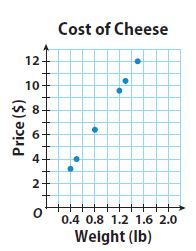Question 13. Is the relationship represented by the graph a function? Justify your reasoning. Use the words “input” and “output” in your explanation, and connect them to the context represented by the graph. _______________ Answer: Yes, the relationship represented by the graph is a function. Each input (weight) in the graph is paired with exactly one output(price). Question 14. Analyze Relationships Suppose the weights and prices of additional wedges of cheese were plotted on the graph. Might that change your answer to question 13? Explain your reasoning. Type below: _______________ Answer: No. As the weight of the cheese will increase, the cost of wedges of cheese will increase as well. Hence, for each input (weight), there would be exactly one output (price). H.O.T. Focus on Higher Order Thinking Question 15. Justify Reasoning A mapping diagram represents a relationship that contains three different input values and four different output values. Is the relationship a function? Explain your reasoning. _______________ Answer: No. Since there are three inputs and four outputs, one of the inputs will have more than one output, hence the relationship cannot be a function. Question 16. Communicate Mathematical Ideas An onion farmer is hiring workers to help harvest the onions. He knows that the number of days it will take to harvest the onions is a function of the number of workers he hires. Explain the use of the word “function” in this context. Type below: _______________ Answer: Number of days = f(number of workers) Explanation: We know that the more the number of workers will be involved in the harvesting of onion, the lesser days it will take to complete. Thus the number of workers becomes the independent variable and the number of days becomes the dependent variable. Here the word function is used to describe that the number of days is dependent on the number of workers. Number of days = f(number of workers) ### Guided Practice – Describing Functions – Page No. 164 Plot the ordered pairs from the table. Then graph the function represented by the ordered pairs and tell whether the function is linear or nonlinear. Question 1. y = 5 − 2x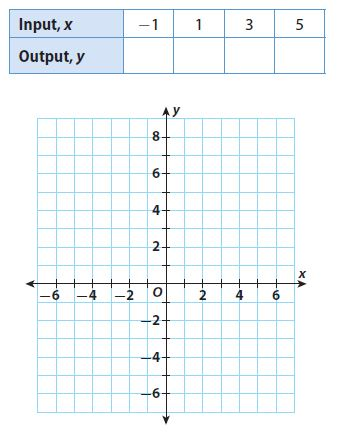_______________ Answer: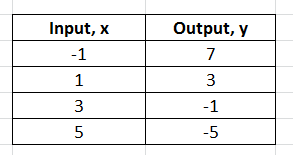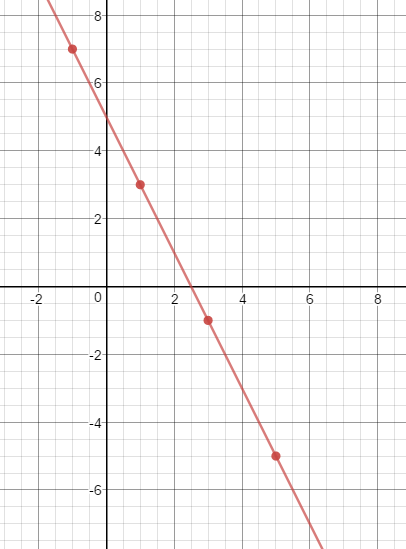Graph of a linear function is a straight line Linear relationship Explanation: Given y = 5 – 2x y = 5 – 2(-1) = 5 + 2 = 7 y = 5 – 2(1) = 5 – 2 = 3 y = 5 – 2(3) = 5 – 6 = -1 y = 5 – 2(5) = 5 – 10 = -5 Question 2. y = 2 − x2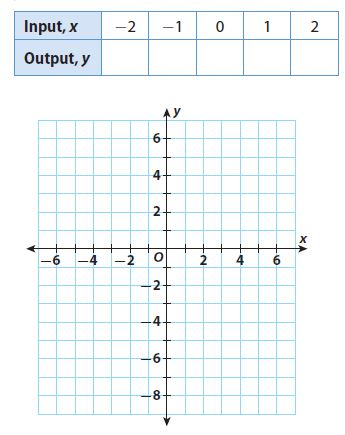_______________ Answer: y = 2 − x2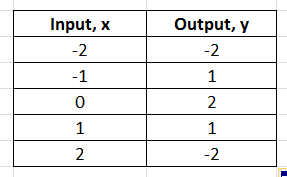Graph the ordered pairs. Then draw a line through the points to represent the solution.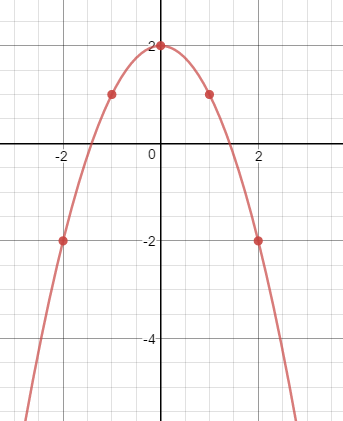Graph of a linear function is not a straight line Non-linear relationship Explanation: y = 2 − x2 y = 2 – 4 = -2 y = 2 – 1 = 1 y = 2 – 0 = 2 y = 2 – 1 = 1 y = 2 – 4 = -2 Explain whether each equation is a linear equation. Question 3. y = x2 – 1 _______________ Answer: The equation is not in the form of a linear equation, hence is not a linear equation. Explanation: Compare the equation with the general linear equation y = mx + b. The equation is not in the form of a linear equation, hence is not a linear equation. Question 4. y = 1 – x _______________ Answer: The equation is in the form of a linear equation, hence is a linear equation. Explanation: Compare the equation with the general linear equation y = mx + b. The equation is in the form of a linear equation, hence is a linear equation. Essential Question Check-In Question 5. Explain how you can use a table of values, an equation, and a graph to determine whether a function represents a proportional relationship. Type below: _______________ Answer: From a table, determine the ratio y/x. If it is constant the relationship is proportional. From a graph, note if the graph passes through the origin. The graph of a proportional relationship must pass through the origin (0, 0). From an equation, compare with general linear form of equation, y = mx + b. If b = 0, the relationship is proportional. ### Independent Practice – Describing Functions – Page No. 165 Question 6. State whether the relationship between x and y in y = 4x – 5 is proportional or nonproportional. Then graph the function.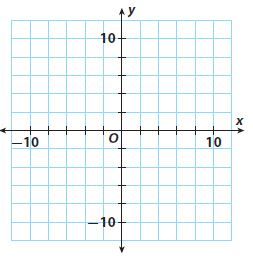_______________ Answer: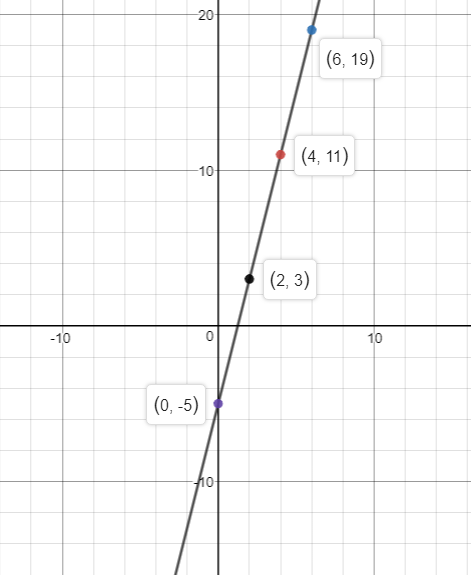Explanation: First, we compare the equation with the general linear equation y = mx + b. y = 4x – 5 is in the form of y = mx + b, with m = 4 and b = -5. Therefore, the equation is a linear equation. Since b is not equal to 0, the relationship is non-proportional. Then, we choose several values for the input x. We substitute these values of x in the equation to find the output y. y = 4x – 5 If x = 0; y = 4(0) – 5 = -5; (0, -5) If x = 2; y = 4(2) – 5 = 3; (2, 3) If x = 4; y = 4(4) – 5 = 11; (4, 11) If x = 6; y = 4(6) – 5 = 19; (6, 19) We graph the ordered pairs and we draw a line through the points to represent the solutions of the function. Question 7. The Fortaleza telescope in Brazil is a radio telescope. Its shape can be approximated with the equation y = 0.013x2. Is the relationship between x and y linear? Is it proportional? Explain. ____________ ____________ Answer: Compare the equation with the general linear equation y = mx + b. The equation is not in the form of a linear equation, hence it is not a linear equation. Since x is squared, it is not proportional. Question 8. Kiley spent$20 on rides and snacks at the state fair. If x is the amount she spent on rides, and y is the amount she spent on snacks, the total amount she spent can be represented by the equation x + y = 20. Is the relationship between x and y linear? Is it proportional? Explain.
____________
____________

x + y = 20
Rewriting the equation
y = 20 – x
Compare the equation with the general linear equation y = mx + b.
It is linear
Since b is not equal to 0, the relationship is not proportional.

Question 9.
Represent Real-World Problems
The drill team is buying new uniforms. The table shows y, the total cost in dollars, and x, the number of uniforms purchased.a. Use the data to draw a graph. Is the relationship between x and y linear? Explain.
____________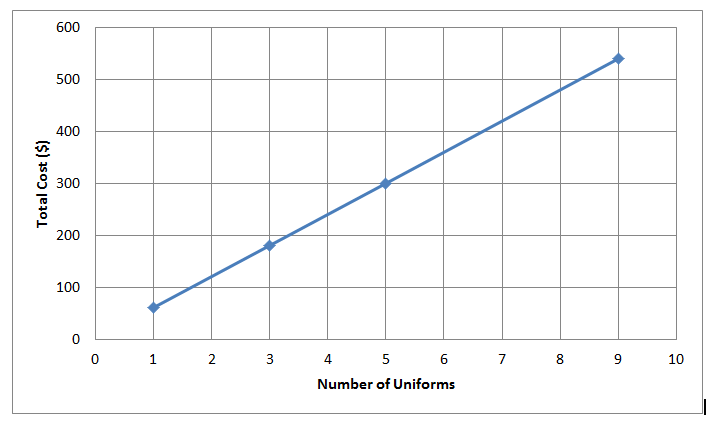The graph of a linear relationship is a straight line.
x and y are linear.

Question 9.
b. Use your graph to predict the cost of purchasing 12 uniforms.
$________ Answer:$720

Explanation:The cost of 12 uniforms is $720 Question 10. Marta, a whale calf in an aquarium, is fed a special milk formula. Her handler uses a graph to track the number of gallons of formula y the calf drinks in x hours. Is the relationship between x and y linear? Is it proportional? Explain.____________ ____________ Answer: The relationship is linear The relationship is proportional Explanation: As the data lies on a straight line, the relationship is linear As the graph passes through the origin, the relationship is proportional ### Describing Functions – Page No. 166 Question 11. Critique Reasoning A student claims that the equation y = 7 is not a linear equation because it does not have the form y=mx + b. Do you agree or disagree? Why?____________ Answer: Disagree; The equation can be written in the form y = mx + b Where m is 0. The graph of the solutions is a horizontal line. Question 12. Make a Prediction Let x represent the number of hours you read a book and y represent the total number of pages you have read. You have already read 70 pages and can read 30 pages per hour. Write an equation relating x hours and y pages you read. Then predict the total number of pages you will have read after another 3 hours. _______ pages Answer: 160 pages Explanation: Let x represent the number of hours you read a book and y represents the total number of pages you have read. You have already read 70 pages and can read 30 pages per hour. m = 30; b = 70 pages y = 30x + 70 x = 3 hrs y = 30(3) + 70 = 160 H.O.T. Focus on Higher Order Thinking Question 13. Draw Conclusions Rebecca draws a graph of a real-world relationship that turns out to be a set of unconnected points. Can the relationship be linear? Can it be proportional? Explain your reasoning. Type below: ______________ Answer: The relationship is linear if all the points lie on the same line. If the relationship is linear and passes through the origin, it is proportional. Question 14. Communicate Mathematical Ideas Write a real-world problem involving a proportional relationship. Explain how you know the relationship is proportional. Type below: ______________ Answer: The amount of money earned at a car wash is a proportional relationship. When there are 0 cars washed,$0 are earned. The amount of money earned increases by the unit cost of a car wash.

Question 15.
Justify Reasoning
Show that the equation y + 3 = 3(2x + 1) is linear and that it represents a proportional relationship between x and y.
Type below:
______________

y + 3 = 3(2x + 1)
y +3 = 6x + 3
y = 6x
As b = 0, it is a Proportional Relationship.

### Guided Practice – Comparing Functions – Page No. 170

Doctors have two methods of calculating maximum heart rate. With the first method, maximum heart rate, y, in beats per minute is y = 220 − x, where x is the person’s age. Maximum heart rate with the second method is shown in the table.Question 1.
Which method gives the greater maximum heart rate for a 70-year-old?
____________ method

Second

Explanation:
y = 220 – x
y = 220 – 70 = 150
Find the slope using two points from the grapgh by
Slope m = (y2 -y1)/(x2 – x1) where (x1, y1) = (20, 194) and (x2, y2) = (30, 187)
Slope m = (y2 -y1)/(x2 – x1) = (187 – 194)/(30 – 20) = -7/10 = -0.7
197 = -0.7(20) + b
y-intercept b = 208
Substituting the value of the slope m and y-intercept in the slope-intercept form. y = mx + b where, m = -0.7 and b = 208.
y = -0.7x +208
x = 70yrs
y = -0.7(70) + 208 = 159
150 < 159
Second method gives the greater maximum heart rate for a 70 year ols.

Question 2.
Are heart rate and age proportional or nonproportional for each method?
____________

For method 1, the relationship is non-propotional.
For method 2, the relationship is non-propotional.

Explanation:
Compare the equation with the general linear equation y = mx + b.
It is linear
Since b is not equal to 0, the relationship is not proportional.

Aisha runs a tutoring business. With Plan 1, students may choose to pay $15 per hour. With Plan 2, they may follow the plan shown on the graph.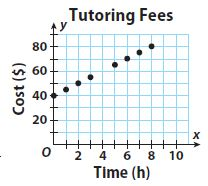Question 3. Describe the plan shown on the graph. Type below: ______________ Answer: Choose two points on the graph to find the slope. Find the slope m = (y2 -y1)/(x2 – x1) m = (60 – 40)/(4 – 0) = 20/4 = 5 Read the y-intercept from the graph: b = 40 Use your slope and y-intercept values to write an equation in slope-intercept form. y = 5x + 40 Plan 2 has an intial cost of$40 and a rate of $5 per hour. Question 4. Sketch a graph showing the$15 per hour option.
Type below:
______________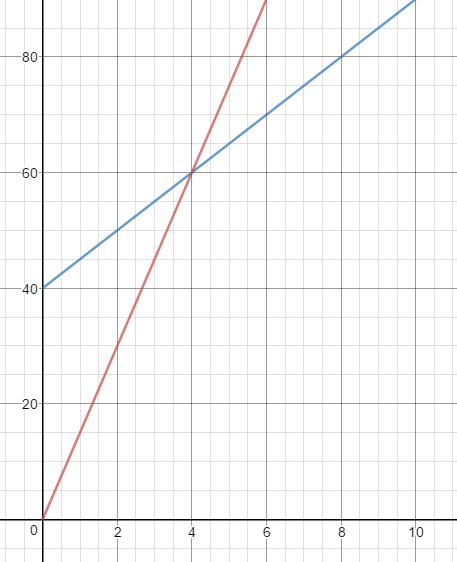Question 5.
What does the intersection of the two graphs mean?
Type below:
______________

The intersection of the two graphs represents the number of hours for which both plans will cost the same,

Question 6.
Which plan is cheaper for 10 hours of tutoring?
______________

Plan 1
y = 15x
x = 10 hrs
y = 15(10) = $150 Plan 2 y = 5x + 40 y = 5(10) + 40 =$90
$150 >$90
Plane 2 is cheaper

Question 7.
Are cost and time proportional or nonproportional for each plan?
Type below:
______________

Comparing with the general linear form of equation y = mx + b. Since b = 0, the relationship is proportional
The cost and time are proportional for Plan 1
Comparing with the general linear form of equation y = mx + b. Since b is not equal to 0, the relationship is proportional
The cost and time are not proportional for Plan 2

Essential Question Check-In

Question 8.
When using tables, graphs, and equations to compare functions, why do you find the equations for tables and graphs?
Type below:
______________

The tables and graphs represent a part of the solution of the function. By writing the equation, any value can be a substitute to evaluate the function and compared it with the equations.

### Independent Practice – Comparing Functions – Page No. 171

The table and graph show the miles driven and gas used for two scooters.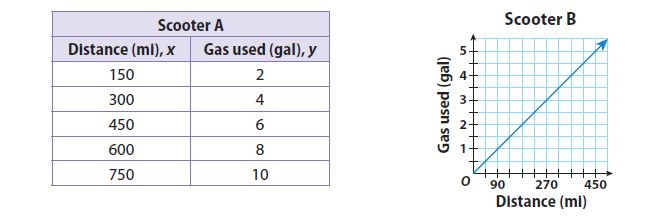Question 9.
Which scooter uses fewer gallons of gas when 1350 miles are driven?
______________

Scooter B uses fewer gallons of gas when 1350 miles are driven

Explanation:
The equation for Scooter A Slope m = m = (y2 -y1)/(x2 – x1) where (x1, y1) = (150, 2) and (x2, y2) = (300, 4)
Slope m = (y2 -y1)/(x2 – x1) = (4 – 2)/(300 – 150) = 2/150 = 1/75
2 = 1/75(150) + b
y-intercept b = 0
Substituting the value of the slope m and y-intercept in the slope-intercept form. y = mx + b where, m = 1/75 and b = 0.
y = 1/75x
x = 1350 miles
y = 1/75(1,350)
y = 18gal
The equation for Scooter B Slope m = m = (y2 -y1)/(x2 – x1) where (x1, y1) = (0, 0) and (x2, y2) = (90, 1)
Slope m = (y2 -y1)/(x2 – x1) = (1 – 0)/(90 – 0) = 1/90
2 = 1/90(90) + b
y-intercept b = 0
Substituting the value of the slope m and y-intercept in the slope-intercept form. y = mx + b where, m = 1/90 and b = 0.
y = 1/90x
x = 1350 miles
y = 1/90(1,350)
y = 15gal
Compare the gallons of gas to drive 1,350 miles
18 > 15
Scooter B uses fewer gallons of gas when 1,350 miles are driven.

Question 10.
Are gas used and miles proportional or nonproportional for each scooter?
______________

The gas used and miles are proportional to both scooters.

Explanation:
Compare with general linear form of an equation, y = mx + b. If b = 0, the relationship is proportional.
The gas used and miles are proportional to both scooters.

A cell phone company offers two texting plans to its customers. The monthly cost, y dollars, of one plan is y = 0.10x + 5, where x is the number of texts. The cost of the other plan is shown in the table.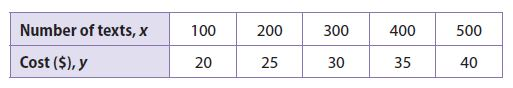Question 11.
Which plan is cheaper for under 200 texts?
______________

Plane 1 is cheaper

Explanation:
Plan 1
y = 0.10x + 5
Subsitute x = 199
y = 0.10(199) + 5 = $24.90 Find the slope using two points from the graph by m = (y2 -y1)/(x2 – x1) where (x1, y1) = (100, 20), (x2, y2) =(200, 25) Substitute the value of m and (x1, y1) = (100, 20), (x2, y2) =(200, 25) Slope m = (y2 -y1)/(x2 – x1) = (25 – 20)/(200 – 100) = 5/100 = 0.05 20 = 0.05(100) + b y-intercept b = 15 Substituting the value of slope (m) and (x, y) in the slope intercept form to find y intercept (b): y = 0.05x + 15 x = 199 y = 0.05(199) + 15 =$24.95
Compare the cost for two plans for text < 200
$24.90 <$24.95
Plane 1 is cheaper

Question 12.
The graph of the first plan does not pass through the origin. What does this indicate?
Type below:
______________

Plan 1
y = 0.10x + 5
The graph that does not pass through the origin indicates that there is a base price of $5 for the plan. Question 13. Brianna wants to buy a digital camera for a photography class. One store offers the camera for$50 down and a payment plan of $20 per month. The payment plan for a second store is described by y = 15x + 80, where y is the total cost in dollars and x is the number of months. Which camera is cheaper when the camera is paid off in 12 months? Explain. ______________ Answer: For first store, the slope interecept form y = mx + b where m = 20 dollars per month and b = 50 dollar. y = 20x + 50 x = 12 months y = 20(12) + 50 =$290
Second store
y = 15x + 80
x = 12 months
y = 15(12) + 80 = $260 Compare the cost of camera if it paid off in 12 months$290 > $260 Camera is cheaper at second store ### Comparing Functions – Page No. 172 Question 14. The French club and soccer team are washing cars to earn money. The amount earned, y dollars, for washing x cars is a linear function. Which group makes the most money per car? Explain.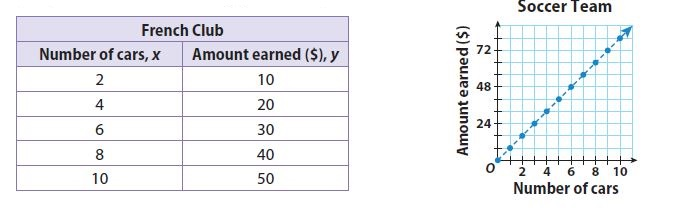______________ Answer: Explanation: Find the slope using two points from the grapgh by Slope m = (y2 -y1)/(x2 – x1) where (x1, y1) = (2, 10) and (x2, y2) = (4, 20) Slope m = (y2 -y1)/(x2 – x1) = (20 – 10)/(4 – 2) = 10/2 = 5 French Club makes$5 per car.
Find the slope using two points from the grapgh by
Slope m = (y2 -y1)/(x2 – x1) where (x1, y1) = (0, 0) and (x2, y2) = (2, 16)
Slope m = (y2 -y1)/(x2 – x1) = (16 – 0)/(2 – 0) = 16/2 = 8
Soccer Club makes $8 per car. Compare the money earned for washing one car$5 < $8 Soccer club makes the most money per car H.O.T. Focus on Higher Order Thinking Question 15. Draw Conclusions Gym A charges$60 a month plus $5 per visit. The monthly cost at Gym B is represented by y = 5x + 40, where x is the number of visits per month. What conclusion can you draw about the monthly costs of the gyms? __________ is more expensive Answer: Gym A is more expensive Explanation: Since the rate per visit is the same, the monthly cost of Gyn A is always more than Gym B. Question 16. Justify Reasoning Why will the value of y for the function y = 5x + 1 always be greater than that for the function y = 4x + 2 when x > 1? Type below: ______________ Answer: y1 = 5x + 1 and y2 = 4x + 2 Subtracting y2 from y1 y1 – y2 = 5x + 1 – (4x + 2) y1 – y2 = x -1 For x>= 1 we get x – 1 >= 0 So y1 – y2 >= 0 or y1 >= y2 Question 17. Analyze Relationships The equations of two functions are y = −21x + 9 and y = −24x + 8. Which function is changing more quickly? Explain. ______________ Answer: y = -21x + 9 y = -24x + 8 y = -24x + 8 is changing more quickly as the absolute value of -24 is greater than the absolute value of -21. ### Guided Practice – Analyzing Graphs – Page No. 176 In a lab environment, colonies of bacteria follow a predictable pattern of growth. The graph shows this growth over time.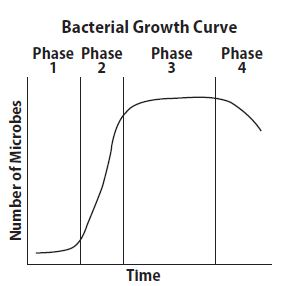Question 1. What is happening to the population during Phase 2? ______________ Answer: For Phase 2, the graph is increasing quickly. This shows a period of rapid growth. Question 2. What is happening to the population during Phase 4? ______________ Answer: In Phase 4, the graph is decreasing, hence the number of bacterias is decreasing. The graphs give the speeds of three people who are riding snowmobiles. Tell which graph corresponds to each situation.Question 3. Chip begins his ride slowly but then stops to talk with some friends. After a few minutes, he continues his ride, gradually increasing his speed. ______________ Answer: Graph 2 Explanation: The slope of the graph is increasing, then it becomes constant and starts increasing again. Graph 2 Question 4. Linda steadily increases her speed through most of her ride. Then she slows down as she nears some trees. ______________ Answer: Graph 3 Explanation: The slope of the graph is increasing and then decreasing. Graph 3 Question 5. Paulo stood at the top of a diving board. He walked to the end of the board, and then dove forward into the water. He plunged down below the surface, then swam straight forward while underwater. Finally, he swam forward and upward to the surface of the water. Draw a graph to represent Paulo’s elevation at different distances from the edge of the pool.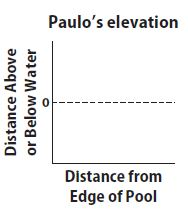Type below: ______________ Answer: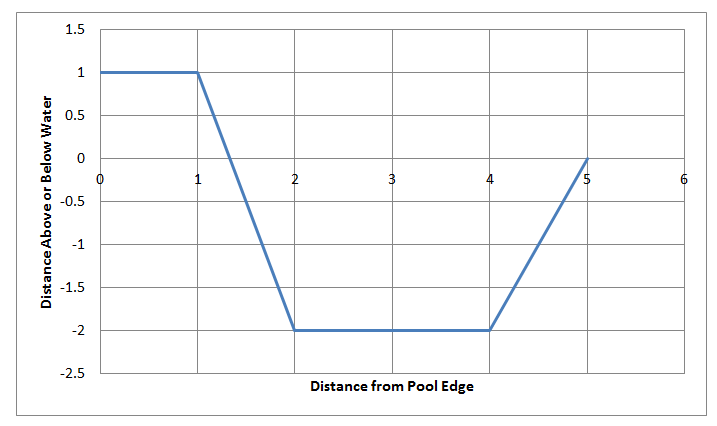### Independent Practice – Analyzing Graphs – Page No. 177 Tell which graph corresponds to each situation below.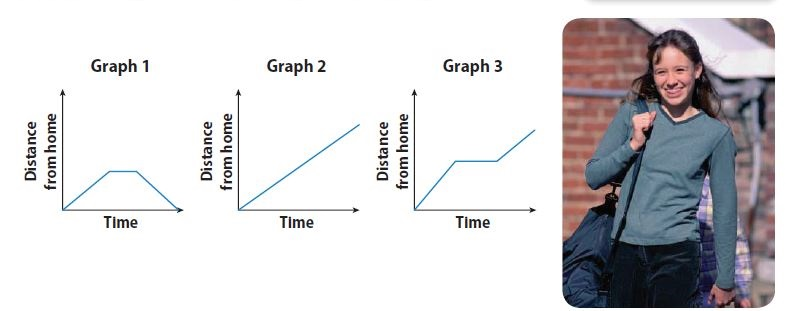Question 6. Arnold started from home and walked to a friend’s house. He stayed with his friend for a while and then walked to another friend’s house farther from home. ______________ Answer: Graph 3 Explanation: The graph increases (as Arnold walks from home to friend’s house), then becomes constant (when he stayed with his friend) and then increases again (when he walk to another friend’s house farther away). Graph 3 Question 7. Francisco started from home and walked to the store. After shopping, he walked back home. ______________ Answer: Graph 1 Explanation: The graph increases (as Francisco walked from home to store), becomes constant (when he shops), and then decreases (as he walked back home) Graph 1 Question 8. Celia walks to the library at a steady pace without stopping. ______________ Answer: Graph 2 Explanation: The graph increases at a constant rate (as Celia walks to library without any stops) Graph 2 Regina rented a motor scooter. The graph shows how far away she is from the rental site after each half hour of riding.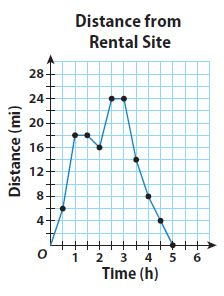Question 9. Represent Real-World Problems Use the graph to describe Regina’s trip. You can start the description like this: “Regina left the rental shop and rode for an hour…” Type below: ______________ Answer: Regina left the rental shop and rode for an hour. She rested for half an hour and then started back. After half an hour, she changed her mind and rode for another half an hour. She rest for half an hour. Then she started back and ranched the rental site in 2 hours. Question 10. Analyze Relationships Determine during which half hour Regina covered the greatest distance. Type below: ______________ Answer: Regina covered the greatest distance between 0.5 to 1hr of the journey. She covered 12 miles. ### Analyzing Graphs – Page No. 178 The data in the table shows the speed of a ride at an amusement park at different times one afternoon.Question 11. Sketch a graph that shows the speed of the ride over time. Type below: ______________ Answer: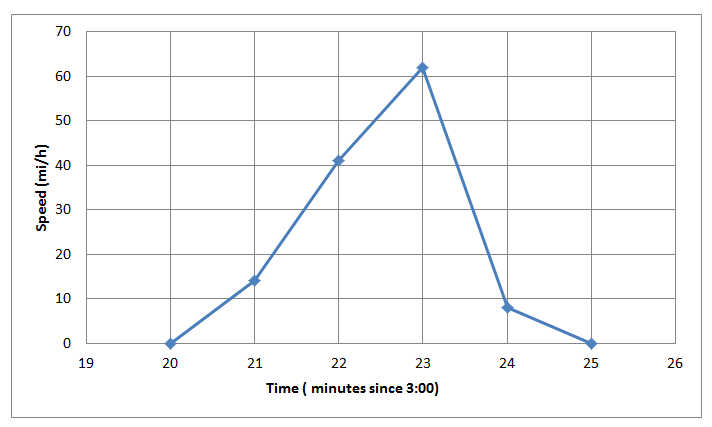Question 12. Between which times is the ride’s speed increasing the fastest? Type below: ______________ Answer: The speed is increasing the fastest during the 3: 21 and 3: 22 Question 13. Between which times is the ride’s speed decreasing the fastest? Type below: ______________ Answer: The speed is decreasing the fastest during the 3: 23 and 3: 24 H.O.T. Focus on Higher Order Thinking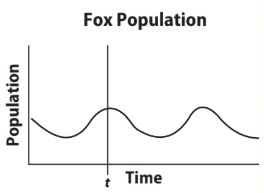Question 14. Justify Reasoning What is happening to the fox population before time t? Explain your reasoning.Type below: ______________ Answer: The population decreases and then increases before time t Question 15. What If? Suppose at time t, a conservation organization moves a large group of foxes to the island. Sketch a graph to show how this action might affect the population on the island after time t.Type below: ______________ Answer: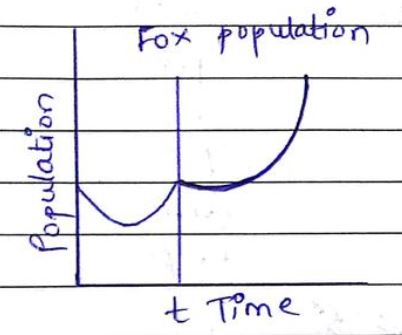Explanation: Population is decreasing at first, then it is increasing rapidly. Question 16. Make a Prediction At some point after time t, a forest fire destroys part of the woodland area on the island. Describe how your graph from problem 15 might change. Type below: ______________ Answer: The population would dramatically decrease if there was a fire due to lack of food supply and good land. ### 6.1 Identifying and Representing Functions – Model Quiz – Page No. 179 Determine whether each relationship is a function. Question 1.__________ Answer: Not a function Explanation: A relationship is a function when each input is paired with exactly one output. The input 5 has more than one output. Not a function Question 2.__________ Answer: Function Explanation: A relationship is a function when each input is paired with exactly one output. Each input is paired with only one output. Function Question 3. (2, 5), (7, 2), (−3, 4), (2, 9), (1, 1) __________ Answer: Not a function Explanation: A relationship is a function when each input is paired with exactly one output. Input 2 has more than one output. Not a function 6.2 Describing Functions Determine whether each situation is linear or nonlinear, and proportional or nonproportional. Question 4. Joanna is paid$14 per hour.
__________
__________

Linear
Proportional

Explanation:
Writing the situation as an equation, where x is the number of hours.
y = 14x
Compare with general linear equation y = mx + b
Linear
Since b = 0, the relationship is proportional.
Proportional

Question 5.
Alberto started out bench pressing 50 pounds. He then added 5 pounds every week.
__________
__________

Linear
Non-proportional

Explanation:
Writing the situation as an equation, where x is the number of hours.
y = 5x + 50
Compare with general linear equation y = mx + b
Linear
Since b is not equal to 0, the relationship is non-proportional.
Non-proportional

6.3 Comparing Functions

Question 6.
Which function is changing more quickly? Explain.__________

Function 2 is changing more quickly.

Explanation:
Find the rate of change for function 1
Rate of Change = (20 – 0)/(0 – 5) = -4
Find the rate of change for function 1
Rate of Change = (6.5 – 11)/(3 – 2) = -4.5
Althogh -4.5 < -4, the absolute value of -4.5 s greater than -4.
Function 2 is changing more quickly.

6.4 Analyzing Graphs

Question 7.
Describe a graph that shows Sam running at a constant rate.
Type below:
______________

The graph would be a straight line

Explanation:
Since Sam is running at a constant rate, distance covered per unit of time remains the same and the relationship is linear and proportional.
The graph would be a straight line

Essential Question

Question 8.
How can you use functions to solve real-world problems?
Type below:
______________

If in the equation the power of x is 1 then it is linear otherwise nonlinear.
In a graph, if the points form a line it is linear if they form a curve it is a nonlinear function.

### Selected Response – Mixed Review – Page No. 180

Question 1.
Which table shows a proportional function?Options:
a. A
b. B
c. C
d. D

c. C

Explanation:
It contains the ordered pair of the origin (0, 0)
Option C represents a proportional relationship.

Question 2.
What is the slope and y-intercept of the function shown in the table?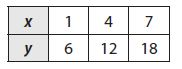Options:
a. m = -2; b = -4
b. m = -2; b = 4
c. m = 2; b = 4
d. m = 4; b = 2

c. m = 2; b = 4

Explanation:
Find the slope using two points from the grapgh by
Slope m = (y2 -y1)/(x2 – x1) where (x1, y1) = (1, 6) and (x2, y2) = (4, 12)
Slope m = (y2 -y1)/(x2 – x1) = (12 – 6)/(4 – 1) = 6/3 = 2
Substituting the value of the slope m and (x, y) to find the slope-intercept form.
12 = 4(2) + b
y-intercept b = 4

Question 3.
The table below shows some input and output values of a function.What is the missing output value?
Options:
a. 20
b. 21
c. 22
d. 23

b. 21

Explanation:
Find the rate of change = (17.5 – 14)/(5 – 4) = 3.5
Since the missing output is corresponding to x = 6 and 3.5 to 17.5 (for x = 5)
Output = 17.5 + 3.5 = 21

Question 4.
Tom walked to school at a steady pace, met his sister, and they walked home at a steady pace. Describe this graph.
Options:
a. V-shaped
b. upside down V-shaped
c. Straight line sloping up
d. Straight line sloping down

b. upside-down V-shaped

Explanation:
The graph would increase at a constant rate and would decrease at a constant rate.
The graph would be the upside-down V-shaped

Question 5.
Linear functions can be used to find the price of a building based on its floor area.Below are two of these functions.
y = 40x + 15,000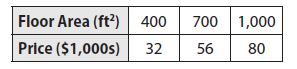a. Find and compare the slopes.
Type below:
____________

Compare the slopes
The slope for the first function is less than the slope of the second function.
y = 40x + 15000
Compare with slope intercept form y = mx + b where m is the slope m = 40
Second function find the slope using given points by Slope m = (y2 -y1)/(x2 – x1) where (x1, y1) = (7, 3) and (x2, y2) = (6, 4)
Slope m = (y2 -y1)/(x2 – x1) = (56000 – 32000)/(700 – 400) = 24000/300 = 80
m = 80

Question 5.
b. Find and compare the y-intercepts.
Type below:
____________

y = 40x + 15,000
Compare with slope-intercept form y = mx + b where m is the slope b = 15000
The second function find the slope using given points by Slope m and (x, y) in the slope-intercept form to fins y-intercept b
y = mx + b where (x, y) = (700, 56000) and m = 80
56000 = 80(700) + b
b = 0
Compare y intercepts
The y-intercept of the first function is greater than the y-intercept of the second function

Question 5.
c. Describe each function as proportional or nonproportional.
Type below:
____________